# Study of Compounds — Hydrogen Chloride

## Exercise 8

#### Question 1

Draw a labelled diagram for the laboratory preparation of hydrogen chloride gas and answer the following.

(a) Name the acid used. Why is this particular acid preferred to other acids?

(b) Give the balanced equation for the reaction.

(c) Name the drying agent used in drying hydrogen chloride gas.

(d) Phosphorus pentoxide and calcium oxide are good drying agents, but they cannot be used to dry hydrogen chloride gas. Why?

(e) Why is direct absorption of HCl gas in water not feasible?

(f) What arrangement is done to dissolve HCl gas in water.

The labelled diagram for the laboratory preparation of hydrogen chloride gas is shown below: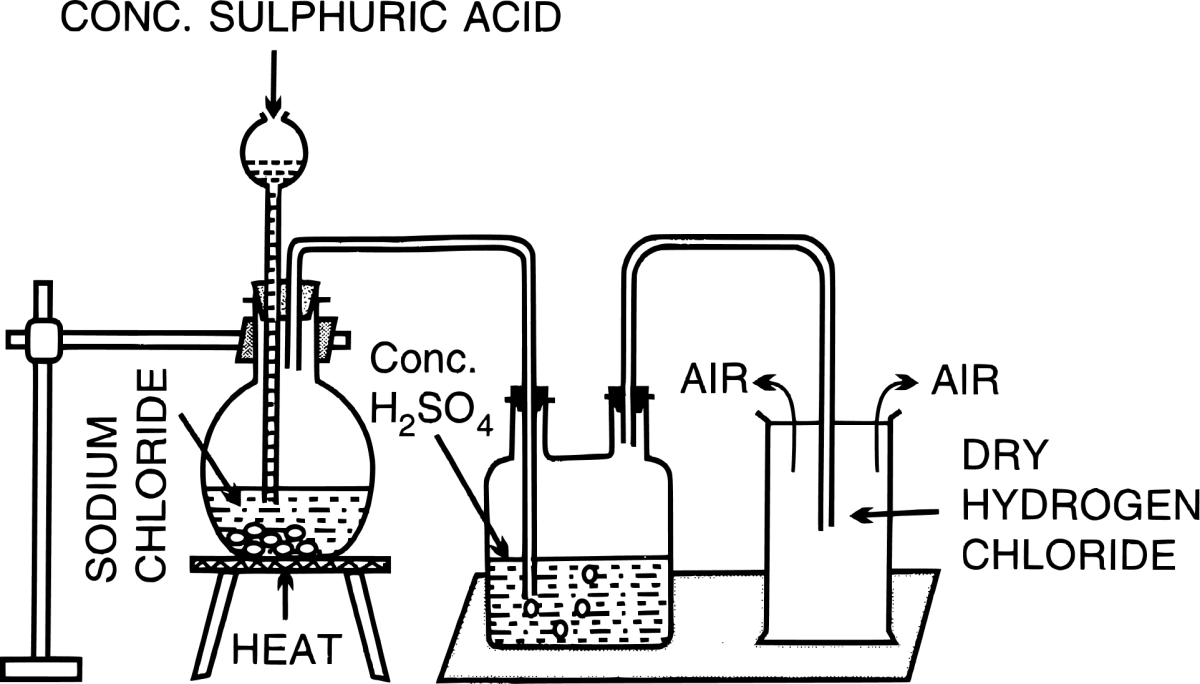(a) Conc. H2SO4 is used for the preparation of hydrogen chloride gas in the laboratory.

As conc. H2SO4 is non-volatile and has a high boiling point, therefore, it displaces the volatile hydrogen chloride from the salt sodium chloride. Hence, conc. H2SO4 is used as a reactant in the laboratory preparation of HCl from sodium chloride.

(b) $\text{NaCl} + \text{H}_2\text{SO}_4 \xrightarrow{\lt 200 \degree\text{C}} \text{NaHSO}_4 + \text{HCl [g.]}$

(c) Conc. H2SO4

Reason — Drying agent used for drying should only remove the moisture and not react with it, hence, conc. sulphuric acid is used as the drying agent.

(d) Phosphorus pentoxide and calcium oxide are good drying agents, but they cannot be used to dry hydrogen chloride gas since they react with it.

2P2O5 + 3HCl ⟶ POCl3 + 3HPO3

CaO + 2HCl ⟶ CaCl2 + H2O

(e) Direct absorption of hydrogen chloride gas in water is not feasible because it causes back suction in the delivery tube.

1. Hydrogen chloride gas is extremely soluble in water.
2. If a delivery tube through which HCl gas is passed is directly immersed in water, the rate of absorption of HCl gas is high and hence a partial vacuum is created in the tube.
3. The pressure outside being higher causes the water to be pushed up into the delivery tube and damages the apparatus. This is called back-suction.

(f) A special funnel arrangement is used to dissolve HCl gas in water as it provides a large surface area for absorption while preventing back-suction. The rim of the funnel is positioned so that it just touches the water in the trough. If back-suction occurs, the water rises up the funnel and the air gap between the rim of the funnel and the water's surface equalizes the pressure inside and outside.

#### Question 2a

Explain why anhydrous HCl is a poor conductor while aqueous HCl is an excellent conductor.

Anhydrous HCl is a gas and does not contain any ions, hence it is a poor conductor of electricity. On the other hand, aqueous HCl (HCl dissolved in water) forms ions in solution, and thus becomes an excellent conductor of electricity.

HCl + H2O ⟶ H3O+ + Cl-

#### Question 2b

Explain why when the stopper of a bottle full of hydrogen chloride gas is opened there are fumes in the air

When hydrogen chloride gas is exposed to air, it gives white fumes, due to the formation of hydrochloric acid on dissolving in atmospheric water vapour.

#### Question 2c

Explain why a solution of hydrogen chloride in water turns blue litmus red and conducts electricity, while a solution of the same gas in toluene

(i) has no effect on litmus, and

(ii) does not conduct electricity.

A solution of hydrogen chloride in water gives ions,

HCl + H2O ⟶ H3O+ + Cl-

These ions are responsible for conducting electricity and turning blue litmus red, due to the presence of hydronium [H3O+] ions which make aqueous solution of HCl acidic in nature.

Toluene is an organic solvent and HCl is a covalent compound. A solution of HCl in toluene contains only molecules and not ions due to which it does not conduct electricity. The absence of hydronium [H3O+] ions make the solution neutral and it does not effect litmus paper.

#### Question 2d

Explain why thick white fumes are formed when a glass rod dipped in NH4OH is brought near the mouth of a bottle full of HCl gas.

When a glass rod dipped in ammonium hydroxide (NH4OH) is brought near the mouth of a bottle full of HCl gas, then it leads to the formation of dense white fumes due to the production of ammonium chloride.

NH4OH + HCl ⟶ NH4Cl + H2O

#### Question 2e

Explain why dry hydrogen chloride gas does not affect a dry strip of blue litmus paper, but it turns red in the presence of a drop of water.

Dry hydrogen chloride gas does not contain any ions. Due to the absence of hydronium [H3O+] ions, it is neutral and does not affect a dry strip of blue litmus paper. On the other hand, in the presence of a drop of water, HCl dissolves in it and dissociates into hydronium [H3O+] ions and chloride ions [Cl-]

HCl + H2O ⟶ H3O+ + Cl-

The presence of hydronium [H3O+] ions makes the aqueous solution acidic and blue litmus turns red.

#### Question 2f

Explain why hydrogen chloride gas is not collected over water.

Hydrogen chloride gas is not collected over water since it is highly soluble in water.

#### Question 3

The given set up in the figure is for the preparation of an acid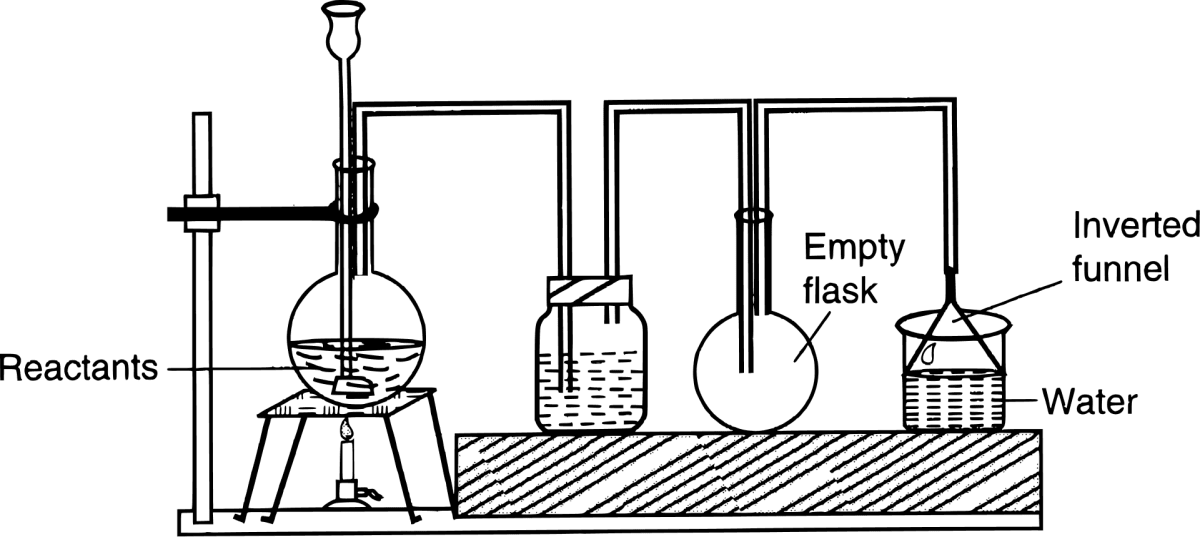(a) Name the acid prepared by this method.

(b) Name the reactants used.

(c) Why an empty flask is used?

(d) What is the drying agent used? Why is this drying agent chosen

(e) What is the role of inverted funnel in the arrangement?

(a) Hydrochloric acid (HCl)

(b) The reactants used are sodium chloride and sulphuric acid.

(c) The empty flask acts as an Anti-Suction device. If back suction takes place then the water gets collected in it and will not reach the generating flask.

(d) The drying agent used is Concentrated Sulphuric acid. It is chosen as drying agent because it does not react with HCl.

(e) The role of the inverted funnel in the arrangement is -

1. It prevents or minimizes back suction of water.
2. It provides a large surface area for absorption of HCl gas.

#### Question 4

(a) (i) Name the experiment illustrated below.

(ii) State the colour of the water that has entered the round-bottomed flask.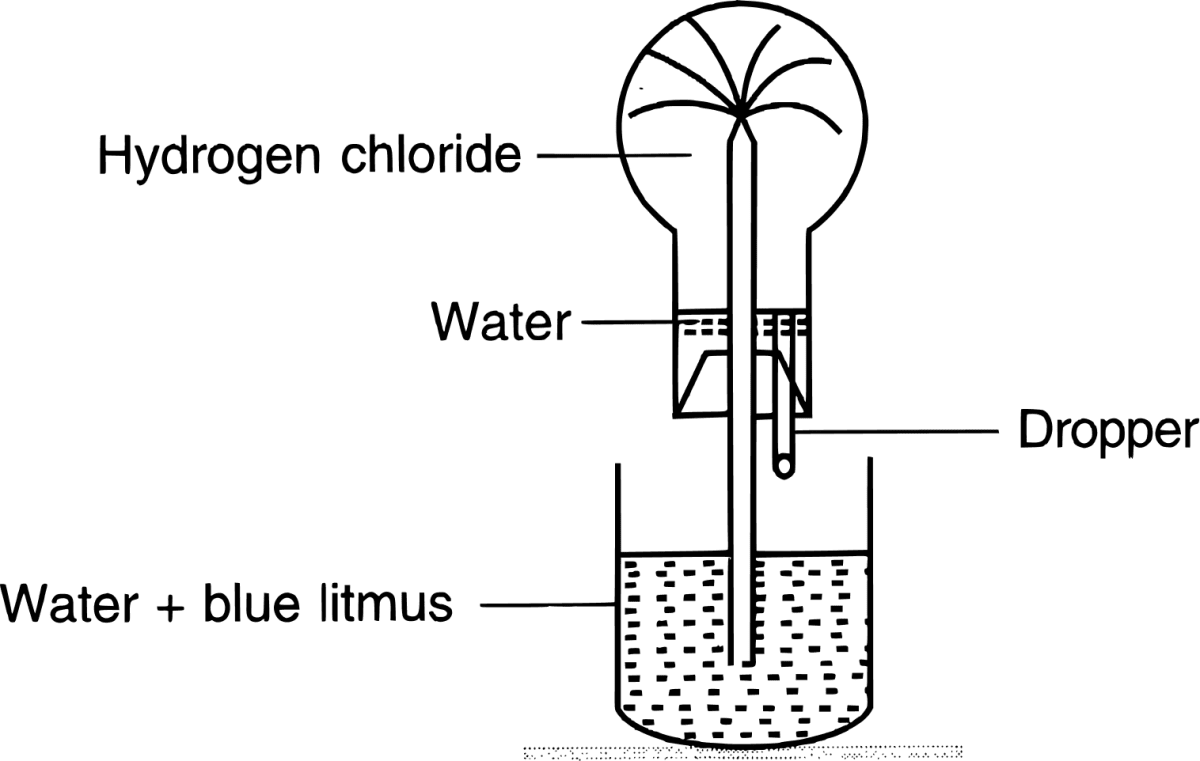(b) What property of hydrogen chloride is demonstrated when it is collected by downward delivery (upward displacement)?

(i) Fountain Experiment.

(b) Red

(c) Hydrogen chloride is 1.28 times heavier than air, hence it is collected by downward delivery [upward displacement].

#### Question 5

(a) Name an element which reacts with hydrogen to form compound which is strongly acidic in water.

(b) Explain why dilute hydrochloric acid cannot be concentrated by boiling beyond 22.2%

(a) Chlorine, It forms HCl which is strongly acidic.

H2 + Cl2 ⟶ 2HCl

(b) Hydrochloric acid forms a constant boiling mixture at 110°C, 22.2% by weight. On boiling further, the mixture evolves out the vapours of both acid and water in the same proportion as in the liquid.

Hence, dilute HCl cannot be concentrated beyond 22.2% by boiling.

#### Question 6

How will you prove that Hydrochloric acid contains

(i) hydrogen

(ii) chlorine.

Write equations for the reactions.

(i) Using an active metal below magnesium

Fe + 2HCl ⟶ FeCl2 + H2

(ii) Using an oxidising agent not containing lead.

MnO2 + 4HCl ⟶ MnCl2 + 2H2O + Cl2

#### Question 7

Name :

(a) a black metallic oxide which reacts with hydrochloric acid to give a coloured solution.

(b) two colourless gases, which when mixed produce a white solid

(c) two gases which chemically combine to form a liquid.

(d) a chloride which is soluble in excess of ammonium hydroxide.

(e) the chemical in which gold can be dissolved.

(f) the experiment which demonstrates that hydrogen chloride is soluble in water.

(g) the gas produced when chlorine water is exposed to sunlight.

(a) Black copper oxide reacts with hydrochloric acid to give a coloured solution.
CuO + 2HCl ⟶ CuCl2 + H2O

(b) Hydrogen chloride and ammonia, when mixed, produce ammonium chloride.
NH3 (g) + HCl (g) ⟶ NH4Cl

(c) Hydrogen and oxygen combine to form water. This can be shown as:
2H2 (g) + O2 (g) ⟶ 2H2O

(d) Silver chloride

(e) Aqua Regia
Aqua regia : 1 part of conc. HNO3 and 3 parts of conc. HCl

(f) Fountain experiment

(g) Oxygen gas

#### Question 8

Solution A reacts with an acid B (which gives greenish yellow gas on reacting with oxidising agents like Pb3O4) to give white precipitate C insoluble in nitric acid but soluble in ammonium hydroxide. Name A, B and C.

A → Silver nitrate
B → Hydrochloric acid
C → Silver chloride

##### Explanation

Solution A is silver nitrate which reacts with acid B i.e., Hydrochloric acid to give a white precipitate C of AgCl which is insoluble in nitric acid but soluble in ammonium hydroxide.

AgNO3 + HCl ⟶ AgCl ↓ + HNO3

AgNO3 + HNO3 ⟶ no reaction

AgCl + 2NH4OH ⟶ [Ag(NH3)2Cl)] + 2H2O

#### Question 9

Complete and balance the following reactions, state whether dilute or conc. acid is used.

(a) NH4OH + HCl ⟶

(b) NaHSO3 + HCl ⟶

(c) Pb(NO3)2 + HCl ⟶

(d) Pb3O4 + HCl ⟶

(a) NH4OH + HCl (dil.)⟶ NH4Cl + H2O

(b) NaHSO3 + HCl (dil.) ⟶ NaCl + H2O + SO2

(c) Pb(NO3)2 + 2HCl (dil.) ⟶ PbCl2+ 2HNO3

(d) Pb3O4 + 8HCl (conc.) ⟶ 3PbCl2 + 4H2O + Cl2

#### Question 10

How will the action of dilute hydrochloric acid enable you to distinguish between the following?

(a) Sodium carbonate and sodium sulphite

(b) Sodium thiosulphate and sodium sulphite.

(a) When Sodium carbonate is treated with dil. HCl, odourless carbon dioxide gas is produced.

Na2CO3 + 2HCl ⟶ 2NaCl + H2O + CO2

However, when sodium sulphite is treated with dil.HCl, sulphur dioxide gas with a suffocating odour (burning smell) is produced.

Na2SO3 + 2HCl ⟶ 2NaCl + H2O + SO2

(b) When sodium thiosulphate is treated with dil. HCl it produces sulphur dioxide gas and yellow sulphur precipitates.

Na2S2O3 + 2HCl ⟶ 2NaCl + S + H2O + SO2

However, S is not produced when sodium sulphite is treated with dil.HCl.

Na2SO3 + 2HCl ⟶ 2NaCl + H2O + SO2

#### Question 11

Give three distinct tests [apart from using an indicator] you would carry out with solution of HCl to illustrate the typical properties of an acid.

(i) Action on metals — Hydrochloric acid reacts with metals above hydrogen in the activity series forming metallic chlorides and evolving hydrogen.

Ca + 2HCl ⟶ CaCl2 + H2

(ii) Action on oxides — Hydrochloric acid reacts with oxides to form salt and water only.

CuO + 2HCl ⟶ CuCl2 + H2O

(iii) With salts of weaker acids — Hydrochloric acid decomposes salts of weaker acids.

Na2CO3 + 2HCl ⟶ 2NaCl + H2O + CO2

#### Question 12

MnO2, PbO2 and red lead react with conc. HCl acid liberates Cl2.

What is the common property being shown by these metal oxides?

It shows that hydrochloric acid (HCl) is oxidized to chlorine (Cl2) by strong oxidizing agents such as MnO2, PbO2 and red lead.

#### Question 13

State which of the two — a solution of HCl in water or in toluene is an electrolyte. Explain.

A solution of hydrogen chloride in water gives ions,

HCl + H2O ⟶ H3O+ + Cl-

These ions are responsible for conducting electricity and turning blue litmus red. Due to the presence of hydronium [H3O+] ions, aqueous solution of HCl is an electrolyte.

Toluene is an organic solvent and HCl is a covalent compound. A solution of HCl in toluene contains only molecules and not ions due to which it does not conduct electricity. The absence of hydronium [H3O+] ions make the solution neutral and does not act as an electrolyte.

#### Question 14

Convert:

(a) Two soluble metallic nitrates to insoluble metallic chlorides using dil. HCl.

(b) Hydrochloric acid to nascent chlorine

(i) Pb(NO3)2 + 2HCl ⟶ PbCl2 + 2HNO3
Hg2(NO3)2 + 2HCl ⟶ Hg2Cl2 + 2HNO3

(ii) HNO3 + 3HCl ⟶ NOCl + 2H2O + 2[Cl] [nascent chlorine]

#### Question 15

A solution of hydrogen chloride in water is prepared.

The following substances are added to separate portions of the solution:

1Calcium carbonate
2Magnesium ribbon
3Manganese(IV) oxide with heating
4Sodium sulphide

Complete the table by writing the gas evolved in case and it's odour.

1Calcium carbonateCO2odourless
2Magnesium ribbonH2odourless
3Manganese(IV) oxide with heatingCl2Pungent smell
4Sodium sulphideH2SRotten egg smell

#### Question 16

State the composition of aqua regia. State which component is the oxidizing agent in aqua regia.

Aqua regia : 1 part of conc. HNO3 and 3 parts of conc. HCl

Nitric acid present in aqua regia oxidizes HCl to chlorine.

#### Question 17

Write an equation for the reactions of hydrochloric acid on :

(a) silver nitrate solution

(b) magnesium foil

(c) caustic soda solution

(d) zinc carbonate

(e) manganese (IV) oxide

(f) copper oxide

(a) Silver nitrate solution
AgNO3 + HCl ⟶ AgCl (white ppt.) + HNO3

(b) Magnesium foil
Mg + 2HCl ⟶ MgCl2 + H2

(c) Caustic soda solution
NaOH + HCl ⟶ NaCl + H2O

(d) Zinc carbonate
ZnCO3 + 2HCl [dil.] ⟶ ZnCl2 + H2O + CO2 [g.]

(e) Manganese (IV) oxide
$\text{MnO}_2 + \underset{\text{Conc.}}{\text{4HCl}} \xrightarrow{\Delta} \text{MnCl}_2 + 2\text{H}_2\text{O} + \text{Cl}_2$

(f) Copper oxide
CuO + 2HCl ⟶ CuCl2 + H2O

#### Question 18

Study the flow chart and give balanced equations with conditions for the conversions A, B, C, D and E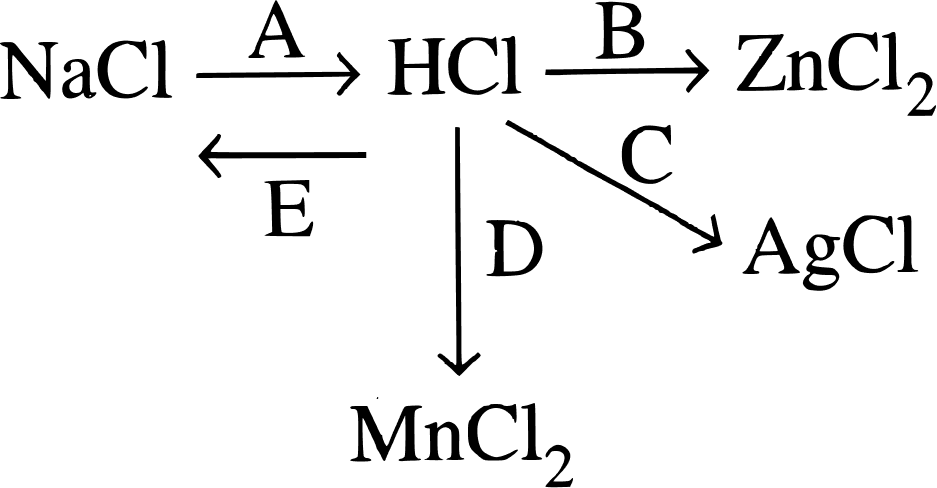The balanced chemical reactions are:

A : $\text{NaCl} + \text{H}_2\text{SO}_4 \xrightarrow{\lt 200 \degree\text{C}} \text{NaHSO}_4 + \text{HCl [g]}$

B : $\text{Zn} + 2\text{HCl ( dil.)} \xrightarrow{\Delta} \text{ZnCl}_2 + \text{H}_2$

C : AgNO3 + HCl ⟶ AgCl (white ppt.) + HNO3

D : $\text{MnO}_2 + \underset{\text{Conc.}}{\text{4HCl}} \xrightarrow{\Delta} \text{MnCl}_2 + 2\text{H}_2\text{O} + \text{Cl}_2$

E : NaHCO3 + HCl ⟶ NaCl + H2O + CO2

#### Question 19

Write the balanced equations for the reaction of dilute hydrochloric acid with each of the following:

(a) Iron

(b) Sodium hydrogen carbonate

(c) Iron (II) sulphide

(d) magnesium sulphite

The balanced equation are :

(a) Iron
Fe + 2HCl (dil.) ⟶ FeCl2 + H2

(b) Sodium hydrogen carbonate
NaHCO3 + HCl ⟶ NaCl + H2O + CO2

(c) Iron (II) sulphide
FeS + 2HCl ⟶ FeCl2 + H2S ↑

(d) Magnesium sulphite
MgSO3 + 2HCl ⟶ MgCl2 + H2O + SO2

#### Question 20

Write the observation:

(a) Lead nitrate solution is mixed with dilute hydrochloric acid and heated.

(b) A small piece of zinc is added to dilute hydrochloric acid.

(a) White precipitate of PbCl2 is formed which is soluble in hot water.
Pb(NO3)2 + 2HCl ⟶ PbCl2 ↓ + 2HNO3

(b) Hydrogen gas is evolved with bubbles and it burns with a pop sound
Zn + 2HCl ⟶ ZnCl2 + H2

#### Question 21

(a) The drying agent used to dry HCl gas is:

A. Conc. H2SO4

B. ZnO

C. Al2O3

D. CaO

(b) When sodium chloride is heated with concentrated sulphuric acid below 200°C, one of the product formed is ............... (Sodium hydrogen sulphate/sodium sulphate/ chlorine).

(a) Conc. H2SO4

Reason — Drying agent used for drying should only remove the moisture and not react with it, hence, conc. sulphuric acid is used as the drying agent.

(b) When sodium chloride is heated with concentrated sulphuric acid below 200°C, one of the product formed is sodium hydrogen sulphate

#### Question 1a(2010)

Aqua regia is a mixture of :

1. Dilute hydrochloric acid and concentrated nitric acid
2. Concentrated hydrochloric acid and dilute nitric acid
3. Concentrated hydrochloric acid [1 part] and concentrated nitric acid [3 parts]
4. Concentrated hydrochloric acid [3 parts] and concentrated nitric acid [1 part]

Concentrated hydrochloric acid [3 parts] and concentrated nitric acid [1 part]

#### Question 1b(2010)

How would you distinguish between dilute HCl and dilute HNO3, by addition of only one solution.

When silver nitrate soln. is added to dil. HCl, curdy white ppt. of silver chloride is formed whereas, there is no reaction when silver nitrate soln. is added to dil. HNO3.

AgNO3 + HCl ⟶ AgCl (white ppt.) + HNO3

AgNO3 + HNO3 ⟶ no reaction

#### Question 1c(2010)

Name two gases which can be used in the study of the fountain experiment. State the common property demonstrated by the fountain experiment ?

Hydrogen chloride gas (HCl) and Ammonia (NH3) gas can be used in the study of the fountain experiment.

High solubility of HCl gas and ammonia gas in water is the common property demonstrated by the fountain experiment.

#### Question 1a(2011)

Choose the correct answer from the choices given:

Hydrogen chloride gas being highly soluble in water is dried by

1. Anhydrous calcium chloride
2. Phosphorous pentoxide
3. Quicklime
4. Conc. sulphuric acid

Conc. sulphuric acid.

Reason — Drying agent used for drying should only remove the moisture and not react with it, hence, conc. sulphuric acid is used as the drying agent.

#### Question 1b(2011)

Write balanced chemical equations.

(i) Sodium thiosulphate is reacted with dilute hydrochloric acid.

(ii) Calcium bicarbonate reacts with dilute hydrochloric acid.

(i) Na2S2O3 + 2HCl ⟶ 2NaCl + S + H2O + SO2

(ii) Ca(HCO3)2 + 2HCl ⟶ CaCl2 + 2H2O + 2CO2

#### Question 1c(2011)

In the laboratory preparation of hydrochloric acid, hydrogen chloride gas is dissolved in water.

(i) Draw a diagram to show the arrangement used for the absorption of HCl gas in water.

(ii) State why such an arrangement is necessary. Give two reasons for the same.

(iii) Write balanced chemical equations for the laboratory preparation of HCl gas when the reaction is :

(A) below 200°C

(B) above 200°C

(i) Below diagrams show the special funnel arrangement used for the absorption of HCl gas in water: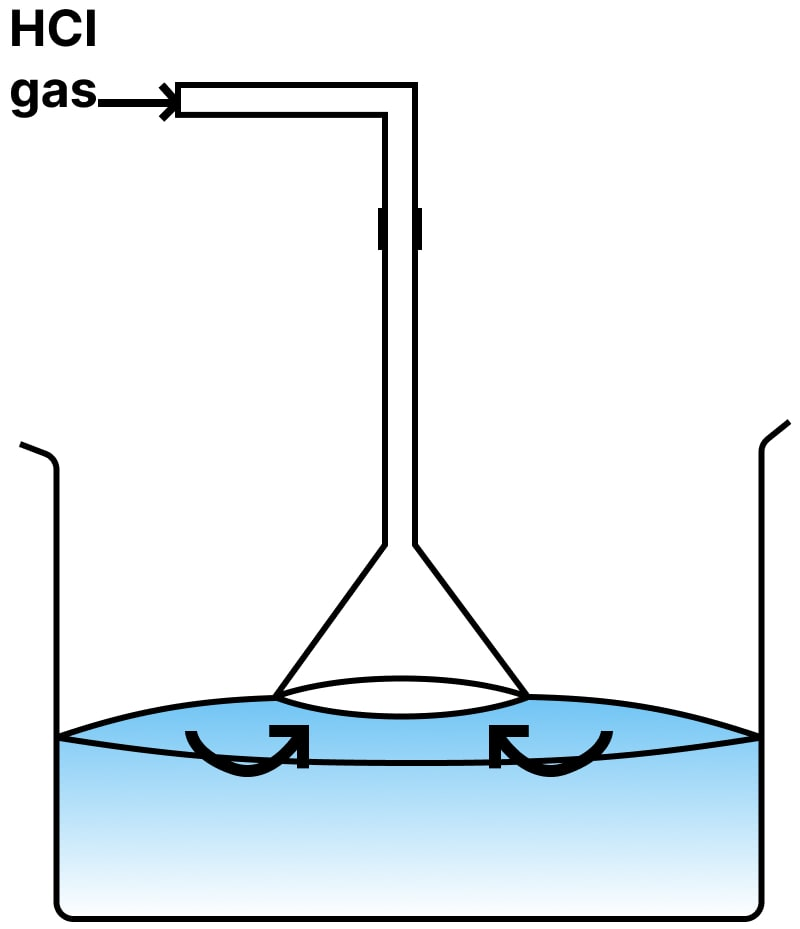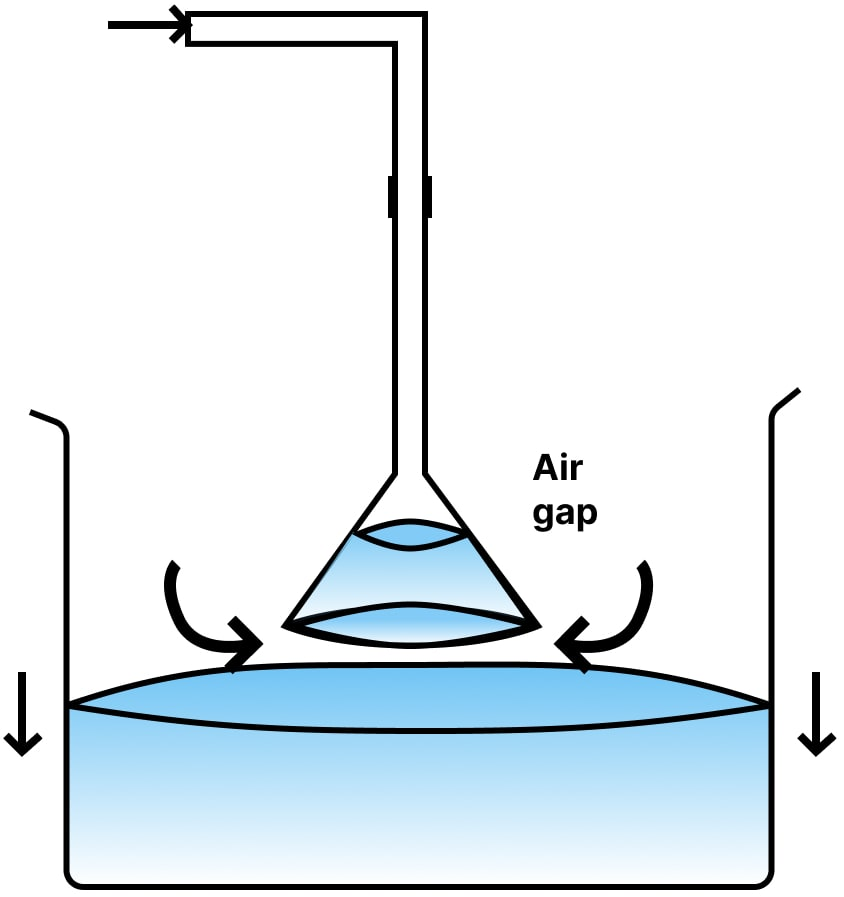(ii) The reasons are :

1. Prevents or minimizes back-suction of water.
2. Provides a large surface area for the absorption of the HCl gas.

(iii) The equations are:

(A) $\text{NaCl} + \text{H}_2\text{SO}_4 \xrightarrow{\lt 200 \degree\text{C}} \text{NaHSO}_4 + \text{HCl [g]}$

(B) $\text{2NaCl} + \text{H}_2\text{SO}_4 \xrightarrow{\gt 200 \degree\text{C}} \text{Na}_2\text{SO}_4 + \text{2HCl [g}]$

#### Question 1a(2012)

Rewrite the correct statement with the missing word/s : Aqua regia contains one part by volume of nitric acid and three parts by volume of hydrochloric acid.

Aqua regia contains one part by volume of conc. nitric acid and three parts by volume of conc. hydrochloric acid.

#### Question 1b(2012)

Give reason for the following :

Hydrogen chloride gas cannot be dried over quick lime.

Quicklime [CaO] is alkaline in nature and it reacts with HCl forming the respective chloride. Hence, it can't be used as a drying agent.

#### Question 1c(2012)

Give a balanced equation for the reaction :

Conc. hydrochloric acid and potassium permanganate solution.

$2\text{KMnO}_4 + 16\text{HCl} \xrightarrow{\Delta} 2\text{KCl} + 2\text{MnCl}_2 + 8\text{H}_2\text{O}+ 5\text{Cl}_2$

#### Question 1d(2012)

Give balanced equations with conditions, if any, for the following conversions

(i) Sodium Chloride ⟶ Hydrogen Chloride

(ii) Hydrogen Chloride ⟶ Iron (II) chloride

(iii) Hydrogen Chloride ⟶ Ammonium chloride

(iv) Hydrogen Chloride ⟶ Lead chloride.

(i) $\text{NaCl} + \text{H}_2\text{SO}_4 \xrightarrow{\lt 200 \degree\text{C}} \text{NaHSO}_4 + \text{HCl [g]}$

(ii) Fe + 2HCl (dil.) ⟶ FeCl2 + H2 [g]

(iii) NH4OH + HCl (dil.)⟶ NH4Cl + H2O

(iv) $\text{PbO}_2 + 4\text{HCl} \xrightarrow{\Delta} \text{PbCl}_2 + 2\text{H}_2\text{O} + \text{Cl}_2$

#### Question 1a(2013)

Identify the gas evolved when :

(i) Potassium sulphite is treated with dilute hydrochloric acid.

(ii) Concentrated hydrochloric acid is made to react with manganese dioxide.

(i) Sulphur dioxide gas

(ii) Chlorine gas
$\text{MnO}_2 + 4\text{HCl} \xrightarrow{\Delta} \text{MnCl}_2 + 2\text{H}_2\text{O} + \text{Cl}_2$

#### Question 1b(2013)

State one appropriate observation when :

(i) Copper sulphide is treated with dilute hydrochloric acid.

(ii) A few drops of dil. HCl are added to AgNO3 soln. followed by addition of NH4OH soln.

(i) A colourless gas having smell of rotten eggs is given off. The gas evolved is H2S

CuS + 2HCl ⟶ CuCl2 + H2S ↑

(ii) Curdy white precipitate of silver chloride [AgCl] is obtained, which is soluble in excess of NH4OH.

AgNO3 + HCl ⟶ AgCl ↓ + HNO3

AgCl + 2NH4OH ⟶ [Ag(NH3)2Cl)] + 2H2O

#### Question 1a(2014)

Fill in the blank from the choices in the bracket :

Quicklime is not used to dry HCl gas because ............... [CaO is alkaline, CaO is acidic, CaO is neutral]

Quicklime is not used to dry HCl gas because CaO is alkaline.

#### Question 1b(2014)

Write the balanced equation for:
Action of dilute hydrochloric acid on sodium sulphide.

Action of dilute hydrochloric acid on sodium sulphide.

Na2S + 2HCl ⟶ 2NaCl + H2S [g.] ↑

#### Question 1c(2014)

Dilute HCl is added to sodium carbonate crystals.

Na2CO3 + 2HCl ⟶ 2NaCl + H2O + CO2

Effervescence of CO2 seen which turns lime water milky

#### Question 1d(2014)

Study the figure given below and answer the questions that follow :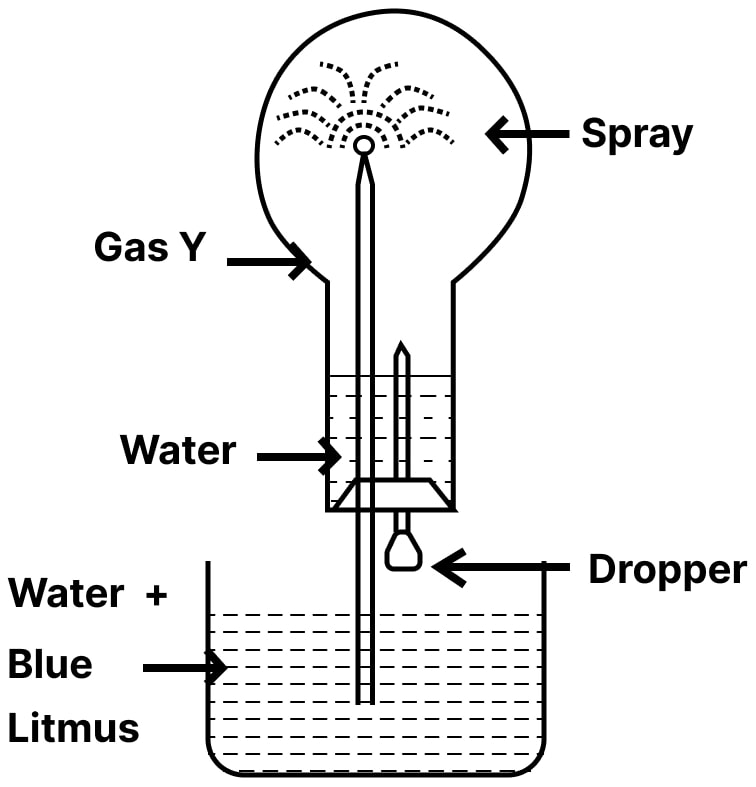(i) Identify the gas Y.

(ii) What property of gas Y does this experiment demonstrate?

(iii) Name another gas which has the same property and can be demonstrated through this experiment.

(i) Dry HCl (Hydrogen chloride) gas is the gas Y

(ii) High solubility of HCl in water

(iii) Ammonia (NH3) gas also demonstrates high solubility in water.

#### Question 1a(2015)

Name the acid which on mixing with silver nitrate solution produces a white precipitate which is soluble in excess of ammonium hydroxide.

Dilute hydrochloric acid

AgNO3 + HCl ⟶ AgCl (white ppt.) + HNO3

#### Question 1b(2015)

Name the gas which produces dense white fumes with ammonia gas.

Hydrogen chloride

NH3 [g] + HCl [g] ⟶ NH4Cl [s]

#### Question 1c(2015)

The following questions pertain to the laboratory preparation of hydrogen chloride gas:

(i) Write the equation for it's preparation mentioning the conditions required.

(ii) Name the drying agent used in the above preparation and give a reason for the choice.

(iii) State a safety precaution taken during the preparation of hydrochloric acid.

(i) The equation for the laboratory preparation of hydrogen chloride gas :

$\text{NaCl} + \text{H}_2\text{SO}_4 \xrightarrow{\lt 200 \degree\text{C}} \text{NaHSO}_4 + \text{HCl [g]}$

(ii) Concentrated sulphuric acid is used as the drying agent used in the above preparation.

Reason — Drying agent used for drying should only remove the moisture and not react with it, hence, conc. sulphuric acid is used as the drying agent. Other drying agents like phosphorous pentoxide (P2O5) and quick lime (CaO) cannot be used since they react with hydrogen chloride gas.

(iii) Temperature is maintained at nearly 200°C as at higher temperatures above 200°C the glass apparatus may crack, fuel is wasted, sodium sulphate formed, forms a hard crust which sticks to the glass and is difficult to remove.

#### Question 1a(2016)

The aim of the Fountain experiment is to prove that:

1. HCl turns blue litmus red
2. HCl is denser than air
3. HCl is highly soluble in water
4. HCl fumes in moist air

HCl is highly soluble in water

#### Question 1b(2016)

Write balanced chemical equation for : Action of hydrochloric acid on sodium bicarbonate.

NaHCO3 + HCl ⟶ NaCl + H2O + CO2

#### Question 1c(2016)

(i) Lead nitrate soln. and the mixture is heated.

(ii) Sodium thiosulphate.

(i) White precipitate of PbCl2 is formed which is soluble in hot water.

Pb(NO3)2 + 2HCl ⟶ PbCl2 ↓ + 2HNO3

(ii) Yellow particles of S precipitate.

Na2S2O3 + 2HCl ⟶ 2NaCl + SO2 + S + H2O

#### Question 1d(2016)

Identify the gas evolved and give the chemical test in each of the following cases.

Dilute hydrochloric acid reacts with:

(i) Iron [II] sulphide.

(ii) Sodium sulphite.